## Want to keep learning?

This content is taken from the The Open University & Persontyle's online course, Advanced Machine Learning. Join the course to learn more.
3.21

# Clustering: Dirichlet Process Mixture Models

In this step we extend Gaussian finite mixture models to the infinite case, and allow the number of clusters/mixtures to be determined by the algorithm.

## Dirichlet Distributions

A Dirichlet distribution with $$k$$ parameters is a distribution over the $$k$$ dimensional ‘standard simplex’. This just means over all values of the $$k$$ variables such:

$\sum_{i=1}^k k=1$

$$0 \leq k_i \leq 1$$, for $$i=1,2,...,k$$

Dirichlet distributions are used in Bayesian statistics to provide a distribution over possible parameters of a corresponding categorical distribution. That is to say, where the parameters of the categorical distribution provide the probability that a variable takes a particular nominal value, the Dirichlet distribution provides a probability distribution over the values of these parameters.

The parameters of a Dirichlet distribution are updated according to observed evidence in a simple count fashion, such that each parameter represents the number of times we have observed the variable take a particular value plus one. Or, if we are encoding expert knowledge rather than updating from data, they represent the ‘pseudo-counts’ that encode knowledge by asking experts to represent their knowledge ‘as if’ they had seen the variable take on different values a certain number of times.

Complete ignorance is represented by all parameters taking the value 1. This results in a uniform distribution over the standard simplex. The distribution peaks at the maximum likelihood estimation of the parameters (the values which maximize the probability of the data seen), which are given by the formula:

$\hat {p}_{ML}(d_i)=\frac{d_i -1}{\sum_{j=1}^k d_j-1}$

And the expected, or MAP (maximum $$a posteriori$$), value of the parameters is given by the formula:

$\hat {p}_{MAP}(d_i)=\frac{d_i}{\sum_{j=1}^k d_j}$

Notice that the MAP estimate builds in some conservativism compared with the ML estimate - we do not immediately jump to certainty based on one observation, for instance.

For example, imagine we wish to model the performance of a football (soccer) team, AI United. The variable, $$V$$, can take the values $$\{win,draw,loss\}$$. We are ignorant about their ability so begin by modeling our knowledge with the Dirichlet distribution:

$Dir(1,1,1)$

This produces the following distribution over the parameters of the categorical distribution over $$V$$: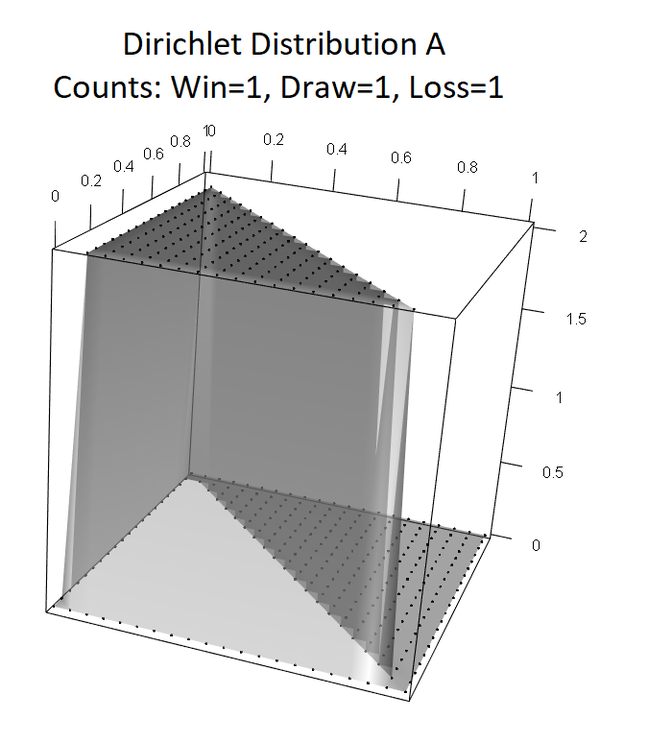It is, as expected, uniform. (N.B. The ‘counts’ include not only the observations but the original ones. So we have not really seen any matches yet.)

Let’s imagine AI United draw the first two games we observe. We update the parameters of the Dirichlet distribution to:

$Dir(1,3,1)$

Which gives this distribution over the parameters of the categorical distribution over $$V$$: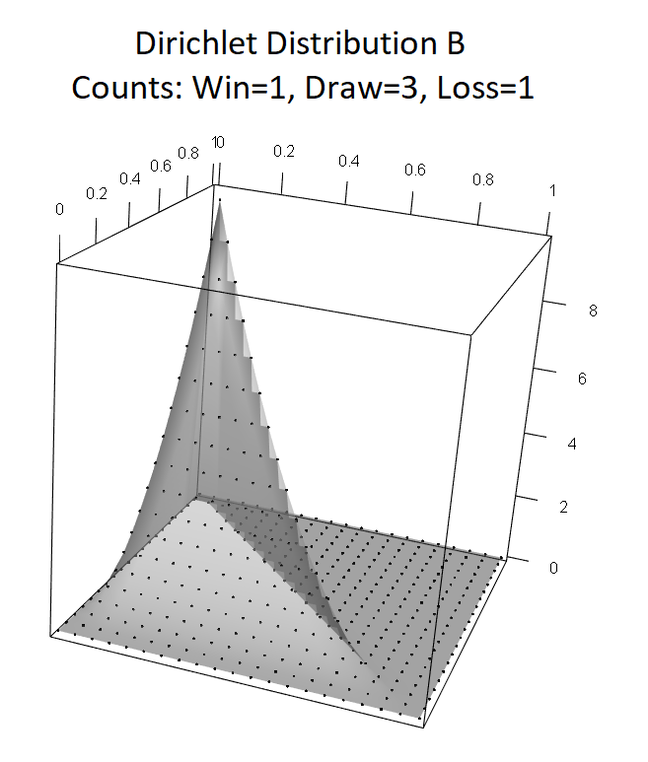Notice that it is peaked in one corner: We can’t rule out the possibility that they will draw all their games yet. In fact, this is the ML estimate.

In their next five games, AI United proceed to win two games, draw one game and lose two games, leading to the updated Dirichlet distribution:

$Dir(3,4,3)$

Giving us this distribution over the parameters of the categorical distribution over $$V$$: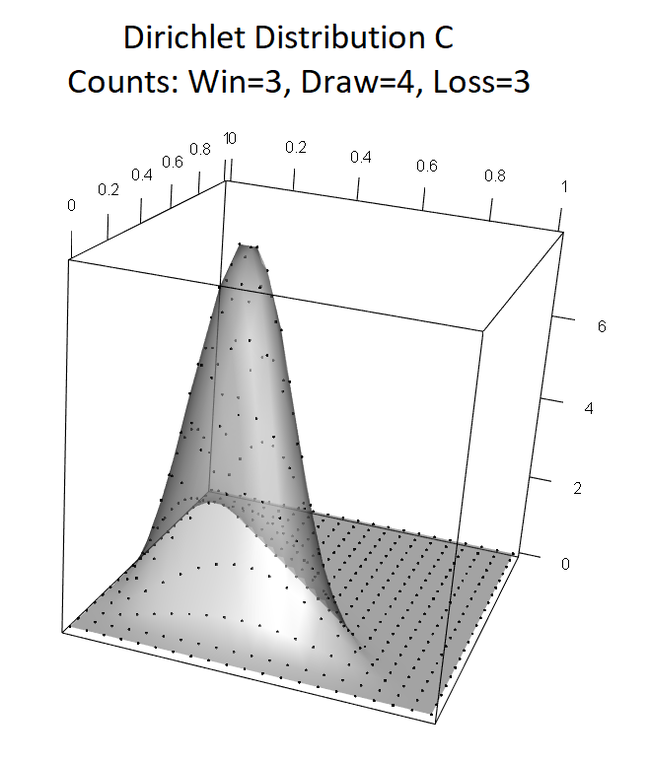Notice it is now rounded, peaking at the maximum likelihood estimates of the parameters given the data (i.e. 2/7 win, 3/7 draw, and, implicitly, 2/7 loss).

Over the next few seasons, AI United proceed to win 27, draw 36 and lose 27 games. The updated Dirichlet is:

$Dir(30,40,30)$

And the distribution over the parameters of the categorical distribution over $$V$$ is: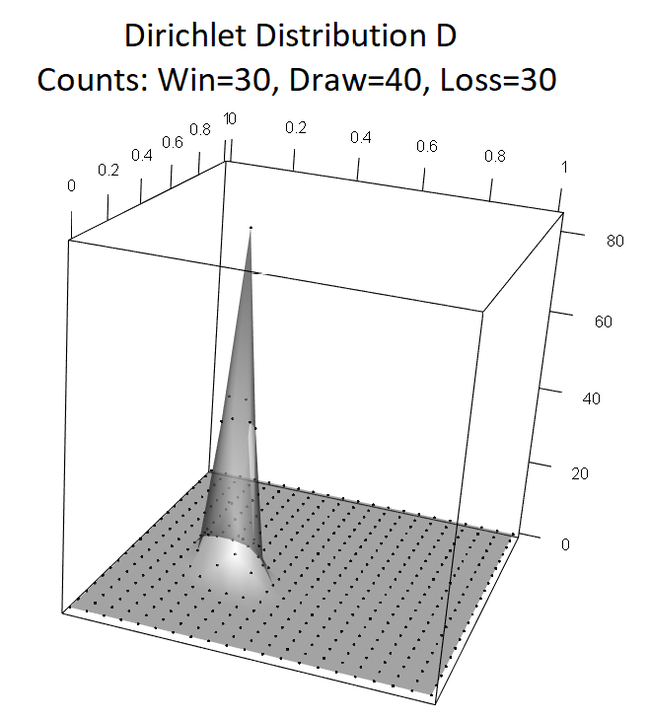What you should notice here is that the MAP estimate remains the same in the last two cases, as the ratio of the different values in the Dirichlet distribution does not change. But the density changes dramatically, becoming must more concentrated around the MAP value as we have more observations. As we have more data, we get more confident in our estimate.

The parameters of the Dirichlet distribution can be positive real number. This allows updating our knowledge on ‘soft’ or probabilistic evidence. For example, we might not know if AI United won or lost their latest game, but know they did not draw. If we assume each alternative equally likely, we can update our Dirichlet distribution to:

$Dir(30.5,40,30.5)$

An important case is when we begin by specifying all parameters as positive reals less than one. In this case, the distribution become concave. For example: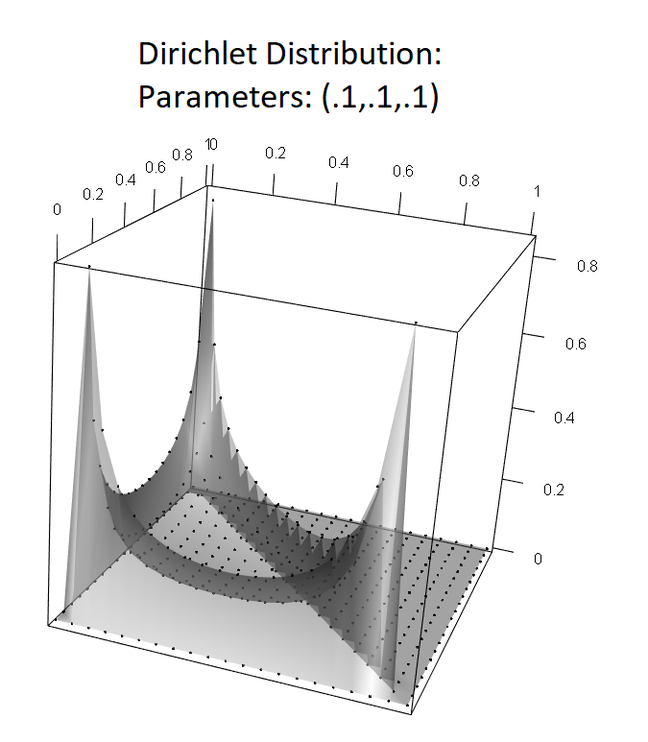This represents a situation where we are confident the variable in question predominantly takes one of its values, but are not sure which one. As soon as we get a single observation the distribution peaks around the catergorical parameter associated with that parameter being near one: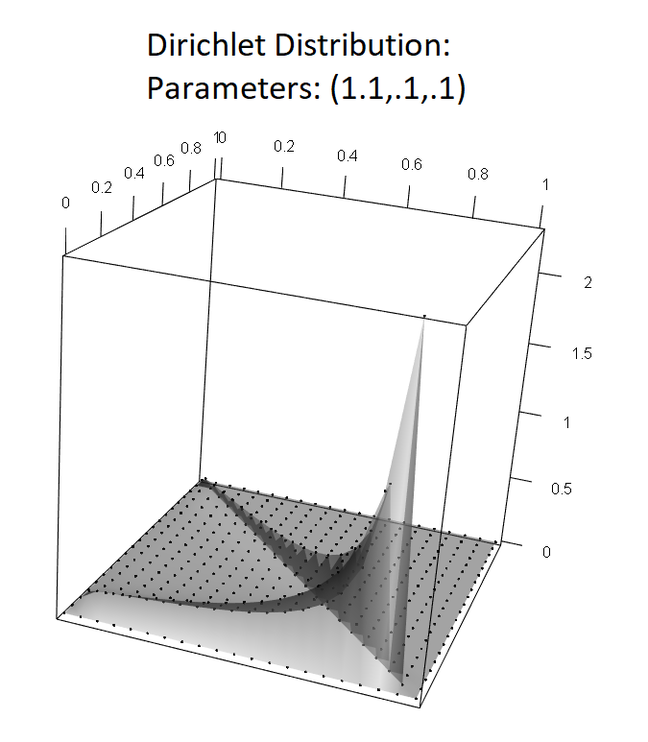We will see this behaviour being exploited in Latent Dirichlet Allocation later.

Finally, it is important to note that the result of updating a Dirichlet distribution, and the total probability of the sequence of data used in the updating, is independent of the order in which the data are presented. The updated Dirichlet, and the probability of seeing AI United win 30 games, draw 40, and lose 30 will be the same if these are presented in random order or wins first, draws second and loses third.

## Dirichlet Processes

A Dirichlet process is a distribution over distributions. It is parameterized by a positive real value, $$\alpha$$, known as the concentration parameter, and a distribution, $$G_0$$.

Assume $$G_0$$ is a standard normal distribution, and $$\alpha$$ is some positive real value. We now explain the process that generates the random distribution, $$G$$ ~ $$DP(\alpha,G_0)$$. We offer the explanations in terms of a process whose colloquial names is the Chinese Restaurant Process.

### Chinese Restaurant Process

Consider a person entering a Chinese restaurant with infinitely many tables, where each table can fit infinitely many guests. Assume that the person will be sit at an occupied table with a probability proportional to the number of people already sitting at that table, and that they will sit at an unoccupied table with a probability proportional to $$\alpha$$. When a table is first occupied, it is assigned a value sampled from $$G_0$$ which is then stamped on the table.

Imagine the state of this restaurant after infinitely many people had entered. Now consider a distribution with probability masses at the points stamped on the tables, these masses proportional to the number of people at each table. Such is the form of the distribution $$G$$ sampled from $$DP(\alpha,G_0)$$.

Let’s consider the initial few customers. Consider the following objects:

$$D_i$$ is a Dirichlet distribution, whose parameters include $$d_*=\alpha$$, and one parameter for each table that currently has customers seated at it, where the value of these parameters is just the number of customers seated at the table.

$$C_i$$ is the MAP of $$D_i$$. It gives the probabilities that a new customer will be seated at a new table or one of the occupied tables.

$$R_i$$ is a set of real numbers keeping track of the real numbers being sampled from $$G_0$$ and stamped on tables.

State 0:

$D_0=[d_*=\alpha]$ $C_0=[c_*=1]$ $R=\emptyset$

Initially, no customers are in the restaurant. The probability that a new customer will be shown to a new table is 1. When a customer enters, they are shown to a new table. A number $$r_1$$ is drawn from $$G_0$$ and stamped on the table. We update our objects.

State 1:

$D_0=[d_*=\alpha,d_1=1]$ $C_0=[c_*=\alpha/(\alpha+1),c_1=1/(\alpha+1)]$ $R={r_1}$

A new customer may now be shown to a new table, or seated at the table with our initial customer. The probabilities of these events are given by $$C$$. Let’s assume that when our second customer enters, they are shown to a new table. Again a number $$r_2$$ is drawn from $$G_0$$ and stamped on the new table. We update our objects.

State 2:

$D_0=[d_*=\alpha,d_1=1,d_2=1]$ $C_0=[c_*=\alpha/(\alpha+2),c_1=1/(\alpha+2),c_2=1/(\alpha+2)]$ $R={r_1,r_2}$

Let’s imagine our third customer is seated with the second. No new real number is drawn. We update our objects:

State 3:

$D_0=[d_*=\alpha,d_1=1,d_2=2]$ $C_0=[c_*=\alpha/(\alpha+3),c_1=1/(\alpha+3),c_2=2/(\alpha+3)]$ $R={r_1,r_2}$

And so it continues. Note some important characteristics:

1. As more customer enter, the chance that they will be assigned to a new table gets less and less.

2. As more customers sit at a table, it become more likely that future customers will sit at the same table. This is known as the ‘rich get richer’ property.

## Clustering with Dirichlet Processes: Dirichlet Process Mixture Models

The Chinese restaurant process gives us a nice metaphor for how we work with Dirichlet processes in clustering. Each datum is a customer, and each table has associated with it a cluster.

Let us assume clusters (or mixtures) are specified by Gaussian distributions. That means that each table has associated with it a mean vector and covariance matrix. Rather than drawing these arbitrarily, we can generate these by the usual methods from the data at the table and calculate the probability that such values would be drawn from the distribution(s) associated with the Dirichlet process.

Given this, we want to find the maximum a posterior assignment of data to clusters. For each datum, this involves:

1. The probability of that datum being associated with particular a particular cluster/table, given from the updated Dirichlet distribution of the assignments of other data (remember that order is unimportant).

2. The probability of the means and covariance matrices (calculated from the current data assigned to that cluster/table) associated with the cluster/table being drawn from the distributions of the Dirichlet process.

3. The probability of the datum being drawn from the Gaussian distribution specified by this mean and covariance.

The distribution in the Dirichlet process for a normal distribution, with parameters mean and covariance, is the normal-inverse gamma. The mean is drawn from the normal distribution, and the covariance from the inverse gamma distribution. But it is more convenient to work with the $$precision$$ rather than covariance matrix, where the precision is the inverse of the covariance. Then the distribution used is the normal-gamma. Again count parameters are used in updating these distributions.

A number of methods can be used to train or perform inference on Dirichlet process mixture models. Analytic solutions to finding the MAP parameters of the mixture model are seldom tractable. Normal methods used include MCMC, EM and variational Bayes. Here we look at variational Bayes.

## Variational Bayes

Let us imagine we know $$p(z)$$ and $$p(x$$|$$z)$$, and want to estimate $$p(z$$|$$x)$$. In variational Bayes, we seek to find the best approximation, $$q(z)$$, to $$p(z$$|$$x)$$. This is done by defining a family of distributions, $$\mathcal{D}_\nu$$, where member of this family are parameterized by a set of parameters, $$\nu$$. In the simplest approach, called mean-field approximation (the name comes from its origin in physics), the $$p$$ is approximated by a collection of independent components:

$$q_\nu(z)=\prod_i q_i(z_i$$|$$\nu_i)$$

The parameters, $$\nu$$, are randomly initialized and then updated in an iterative process so as reduce the KL-divergence of $$p(z$$|$$x)$$from $$q(z)$$, $$D_{KL}(q(z),p(z$$|$$x))$$ (the KL-divergence is a measure of the divergence of one distribution from another, see the aside below for more information). The result of this process is the $$\hat{q}(z) \in \mathcal{D}_\nu$$, which will either be the distribution in $$\mathcal{D}_\nu$$ that has least KL-divergence with the target $$p(z$$|$$x)$$ distribution, or a local optima of the minimization process.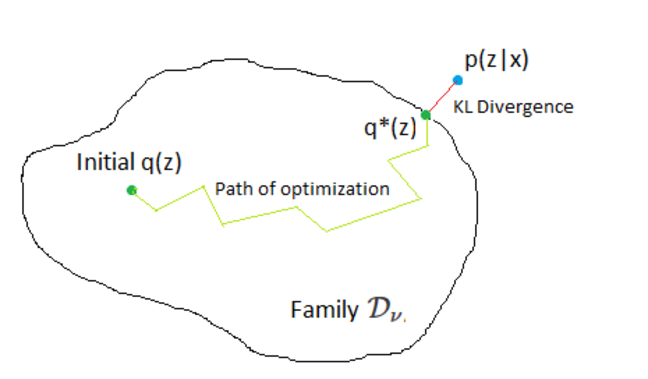There are a number of algorithms that can be used for the iterative optimization process, the most common of which is called coordinate ascent mean-field variational inference (CAVI). This algorithm is very similar to the EM algorithm, except that now the distribution being optimized is the approximation $$q(z$$|$$\nu)$$, while the known distributions $$p(z)$$ and $$p(x$$|$$z)$$ are used to work out how these updates should proceed. The math involved is complex, and we leave the interested student to research the algorithm further themselves.